# Types of Function Arguments in Python

## Types of Function Arguments in Python with Examples

In this article, I am going to discuss Types of Function Arguments in Python with Examples. Please read our previous article where we discussed Functions in Python with examples. At the end of this article, you will understand the following pointers in detail which are related to python Function Arguments.

1. Types of Function Arguments in Pythons
2. Positional Arguments
3. Keyword Arguments in Python
4. Default Arguments in Python Function
5. Variable Length Arguments in Python
6. keyword variable-length argument
7. Function vs Module vs Library
##### TYPES OF ARGUMENTS IN PYTHON FUNCTIONS

Argument: An argument is a variable (which contains data) or a parameter that is sent to the function as input. Before getting into argument types, let’s get familiar with words formal and actual arguments.

1. Formal arguments: When a function is defined it (may) has (have) some parameters within the parentheses. These parameters, which receive the values sent from the function call, are called formal arguments.
2. Actual arguments: The parameters which we use in the function call or the parameters which we use to send the values/data during the function call are called actual arguments.
###### Example: formal and actual function arguments in python (Demo15.py)
```def sum(a, b):
c = a + b            # a and b are formal arguments
print(c)
# call the function
x = 10
y = 15
sum(x, y)            # x and y are actual arguments```

Output: 25

##### Types of Arguments in Pythons:

In python, depending on the way or format we send the arguments to the function, the arguments can be classified into four types:

1. Positional arguments
2. Keyword arguments
3. Default arguments
4. Variable-length arguments
5. keyword variable-length argument
##### Positional Arguments in Python:

If there are three arguments (formal arguments) in the function definition, then you need to send three arguments(actual arguments) while calling the function. The actual arguments will be received by the formal arguments in the same order as in the function call. Let’s understand this through an example.

In demo16.py the actual arguments 10, 20 will be received in the same order sent i.e 10 will go into variable ‘x’, and 20 will go into the variable ‘y’. If we send the arguments as 20,10 then 20 will go into x and 10 will go into y. The number of arguments and position of arguments should be matched, otherwise, we will get errors as shown in demo17.py

###### Example: Positional arguments (Demo16.py)
```def sub(x, y):
print(x-y)
# calling function
sub(10, 20)```

Output: -10

###### Example: Positional arguments (Demo17.py)
```def sub(x, y):
print(x-y)
# calling function
sub(10, 20,30)```

Output: TypeError: sub() takes 2 positional arguments but 3 were given

##### Keyword Arguments in Python:

In the function call, if we send the values using keys then they are called keyword arguments. Here, keys are nothing but the names of the variables. In a way, we can say that keyword arguments are arguments that recognize the parameters by the name of the parameters. Let’s understand it by the example demo18.py where the name of the function is cart() and parameters are item and price can be written as:

def cart(item, price):

At the time of calling this function, we must pass two values and we can write which value is for what by using the name of the parameter, for example,

cart(item=”bangles”, price=20000)
cart(item=”handbag”, price=100000)

item and price are called keywords in this scenario. Here we can change the order of arguments.

###### Example: Keyword arguments (Demo18.py)
```def cart(item, price):
print(item, "cost is :" ,price)

cart(item="bangles", price=20000)
cart(item="handbag", price=100000)
cart(price=1200, item="shirt")```
###### Output: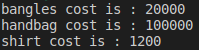###### Example: Keyword arguments (Demo19.py)
```def details(id, name):
print("Emp id is: ",id)
print("Emp name is: ",name)

# calling function
details(id=1, name="Balayya Babu")
details(id=2, name="Chiru")```
###### Output: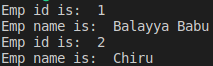Note: We can use both positional and keyword arguments simultaneously. But first, we must take positional arguments and then keyword arguments, otherwise, we will get syntax errors as shown in demo21.py

###### Example: Positional and keyword arguments in Python (Demo20.py)
```def details(id, name):
print("Emp id is: ",id)
print("Emp name is: ",name)

# calling function
details(1, name="Anushka")```
###### Output: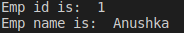###### Example: Positional and keyword arguments in Python (Demo21.py)
```def details(id, name):
print("Emp id is: ",id)
print("Emp name is: ",name)

# calling function
details(name="Anushka",1)```

Output: SyntaxError: non-keyword arg after keyword arg

##### Default Arguments in Python Function with Examples:

In the function definition, while declaring parameters, we can assign some value to the parameters, which are called default values. Such default values will be considered when the function call does not send any data to the parameter. Let’s take the function from demo18.py as an example. There, the function cart has parameters as items and price. Let’s say the price of many items is 20 rupees. In this case, we can set the price param to the default value of 20 rupees

def cart(items, price=20):

In the above example items have no default value, so we should provide. price has a default value of 20. Still, if we provide the value at the time of calling then default values will be overridden with passed value.

###### Example: Default Arguments in Python (Demo22.py)
```def cart(item, price=20):
print(item, "cost is :" ,price)

cart(item="pen")
cart(item="handbag", price=10000)
cart(price=500, item="shirt")```
###### Output: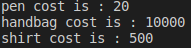Note: If we are not passing any value, then only the default value will be considered. While defining a function, after default arguments we should not take non-default arguments.

###### Example: Default arguments (Demo23.py)
```def cart(item=1, price):
print(item, "cost is :" ,price)

cart(item="pen")
cart(item="handbag", price=10000)
cart(price=500, item="shirt")```

Output: SyntaxError: non-default argument follows default argument

##### Variable Length Arguments in Python Function:

Sometimes, the programmer does not know how many values need to pass to function. In that case, the programmer cannot decide how many arguments to be given in the function definition. Therefore, we use variable-length arguments to accept n number of arguments.

The variable-length argument is an argument that can accept any number of values. The variable-length argument is written with a ‘*’ (one star) before the variable in the function definition.

###### Syntax: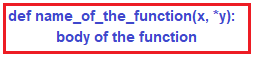x is a formal argument, *y is a variable-length argument. Now we can pass any number of values to this *y. Internally the provided values will be represented in the tuple.

###### Example: Variable-length argument in python (Demo24.py)
```def total_cost(x, *y):
sum=0
for i in y:
sum+=i
print(x + sum)

#calling function
total_cost(100, 200) #valid
total_cost(110, 226, 311) #valid
total_cost(11,) #valid```
###### Output: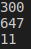##### keyword variable-length argument (**variable) in Python:

Just as variable length arguments, there are keyword variable length arguments that are n key-value pairs. The syntax is given below.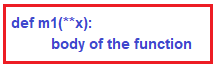**x represents as keyword variable argument. Internally it represents a dictionary object. A dictionary stores the data in the form of key-value pairs.

###### Example: keyword variable length argument (**variable) in Python (Demo25.py)
```def print_kwargs(**kwargs):
print(kwargs)

print_kwargs(id=1, name="Nireekshan", qualification="MCA")```
###### Output: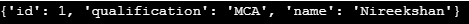###### Example: keyword variable-length argument (**variable) (Demo26.py)
```def m1(**x):
for k, v in x.items():
print(k,"=",v)

m1(a=10, b=20, c=30)
m1(id=100, name="Subbalaxmi")```
###### Output: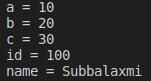##### Function vs Module vs Library in Python:
1. A group of lines with some name is called a function
2. A group of functions saved to a file is called Module
3. A group of Modules is nothing but Library

1.